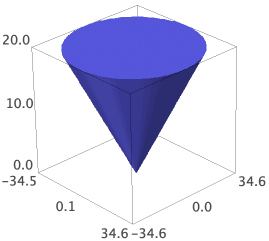# How to create a 3D plot of a parametric equation that requires 3 variables?

I'm try to plot (one nappe of) a cone using the parametric formula (from https://en.wikipedia.org/wiki/Cone#Eq...) :where s , t , u range over [ 0 , θ ), [ 0 , 2 π ) , and [ 0 , h ], respectively.

I tried:

parametric_plot3d((u*tan(s)*cos(t),u*tan(s)*sin(t),u),(u,0,20),(s,0,60*(pi/180)),(t,0,2*pi) )


but it took the third range as plot_points.

Then I tried:

parametric_plot3d((u*tan(t/6)*cos(t),u*tan(t/6)*sin(t),u),(u,0,20),(t,0,2*pi) )


attempting to let t do double duty as s also. It plots, but produces:Which is pretty, but no cigar.

How can I achieve my goal?

Update: I found another formulation of the equation on the web and tried:

parametric_plot3d( ( sin(x*arctan(y/x))*sin(y*arctan(y/x)), sin(x*arctan(y/x))*cos(y*arctan(y/x)), sin(x*arctan(y/x)) ), (x,-pi,pi), (y,-pi,pi) )


And got:Ditto on the absence of stoggies.

edit retag close merge delete

Sort by » oldest newest most votedThe first parametrization is the parametrization of a solid cone.

To plot it, you can plot the boundary, which is where $s=\theta$ or $u=h$:

sage: h = 20
sage: theta = pi/3
sage: side = parametric_plot3d((lambda t, u: u*tan(theta)*cos(t),
....:                           lambda t, u: u*tan(theta)*sin(t),
....:                           lambda t, u: u), (0, 2*pi), (0, h))
sage: base = parametric_plot3d((lambda s, t: h*tan(s)*cos(t),
....:                           lambda s, t: h*tan(s)*sin(t),
....:                           lambda s, t: h), (0, theta), (0, 2*pi))
sage: side + basemore

I actually only need the conical surface. Thankyou for factoring that out for me.